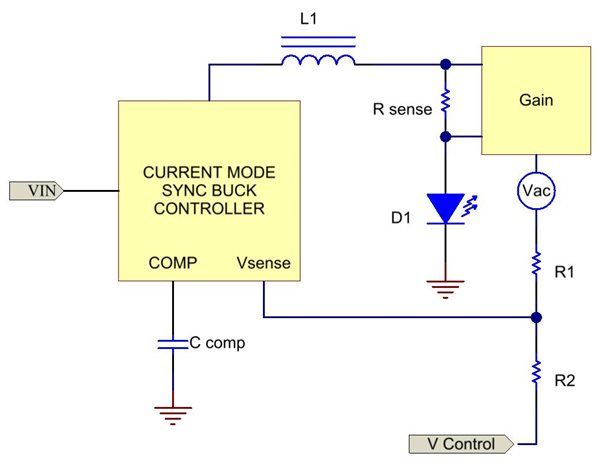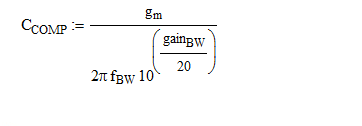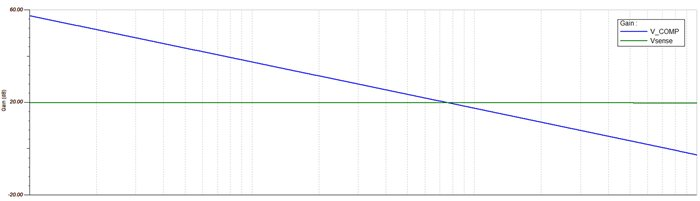# Power Tips: Current Mode Control Makes Compensating a Buck LED Regulator Easy

Other Parts Discussed in Post: TPS54218

Regulating the current in an LED from a higher input voltage is most efficiently done with a sync-buck regulator.  This can be easily achieved with an integrated FET, peak current-mode controller.In peak current-mode control, the COMP voltage (or error signal as it is often called), directly controls the peak inductor current.  This makes the inductor appear as a current source, as its impedance variations have little effect on the current amplitude.  A major benefit of using a peak current-mode controller such as the TPS54218, is that this nearly eliminates the inductor’s frequency response from the control loop gain.

The block diagram below details a sync-buck converter where the LED and sense resistor are in series with the inductor.  In this application, the inductor’s full ripple current flows through the LED.  If less ripple current is desired, simply increasing the inductance value or placing a capacitor in parallel with the LED will work.  Regardless of whether there’s an output capacitor or not, it’s important to have the current sense resistor in series with the inductor current.  Using this as the feedback element makes the controller’s power stage gain relatively flat and the compensation simple.  The dominant pole typically seen in a voltage-mode power stage gain and set by the inductor and output capacitor (or the LED’s ac impedance) disappears.  The combination of current-mode control and current regulation works like magic!In order to decrease power dissipation and maintain high efficiency, a Gain block was added to the sense resistor’s signal.  Resistor divider R1/R2 allow for external adjustment of the LED current by varying the Vcontrol signal level.  For a typical converter, the power stage gain is generally measured from the COMP pin to the Vsense input, and excludes the internal error amplifier.  In the circuit below, this is the summation of gains from the sense resistor-to-COMP, gain block, and the R1/R2 divider.  The plot below labeled Vsense shows a simulation of these totaled gains.  There is little change in this response versus frequency, due to the use of current feedback and current-mode control.  A similar response is possible without the Gain block and the R1/R2 divider.  This could be obtained if the sense resistor were placed on the ground side of the LED and its current sense signal connected directly to Vsense.

To complete the loop gain calculation, only the internal error amplifier response is needed and simply sums with the power stage gain, Vsense.  Since the internal error amp of the TPS54218 is a tranconductance amplifier, with a gm of 225uS (V/A), a capacitor from COMP to ground is all that is necessary for the compensation.  This results in a total loop gain (V_COMP) similar to the one plotted below.  The capacitance value is selected by first choosing the error amplifier gain necessary to obtain a desired bandwidth.  The following equation then calculates the capacitor value, where gainBW is the desired error amplifier gain in dB at the chosen crossover frequency.  Alternatively, the error amplifier’s gain can be determined by its gm divided by the capacitor’s impedance at the frequency of interest.Current-regulated current-mode control simplifies stabilizing the control loop by eliminating the dominate pole in the power stage and reduces the number of compensation components.  Keep in mind that this is a first order approximation for the loop gain and that slope compensation can introduce a pole in the power stage, which can roll off the power stage gain at higher frequencies.  So it’s a good idea not to push for too high of a bandwidth and to always verify the results in the lab.